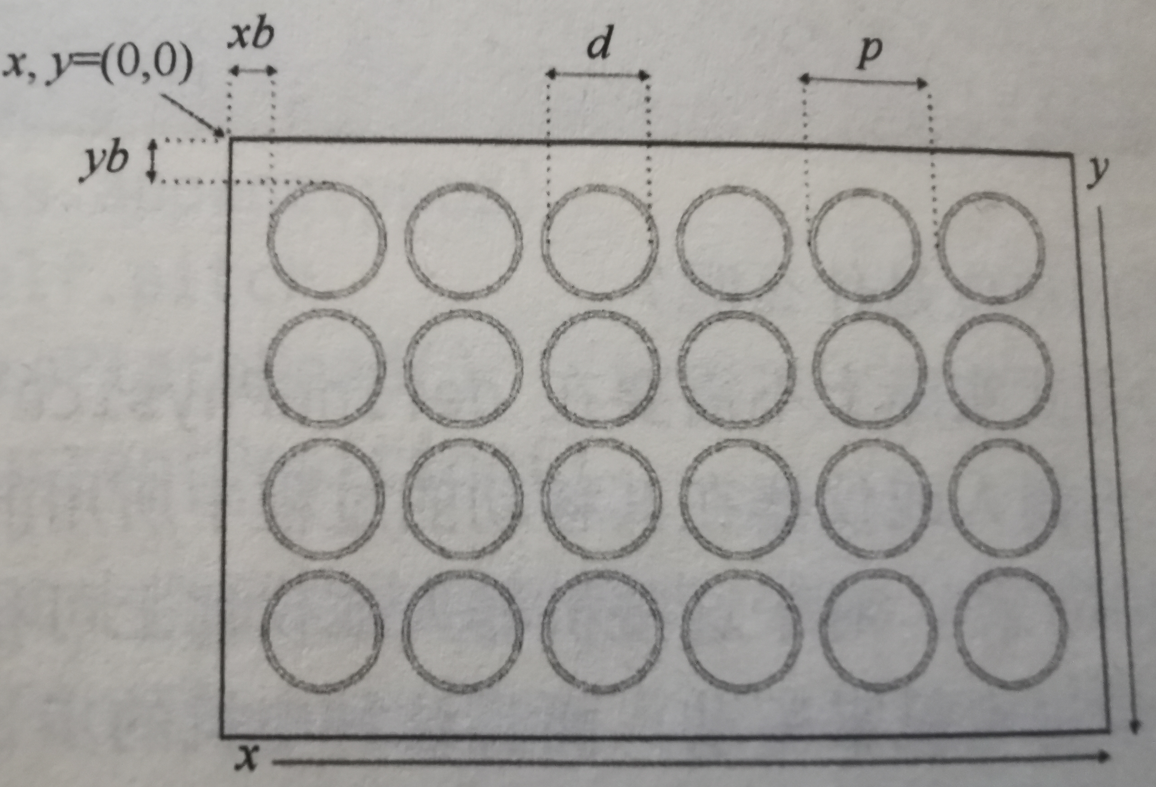V1

2023/02/04阅读：12主题：默认主题

# 1.建立孔板的物理坐标系图1 96孔板的物理布局

``    def definePhysicalMap(self, width, height, xBorder, yBorder, diameter, pitch, stepsize):        self.width = width        self.height = height        self.xBorder = xBorder        self.yBorder = yBorder        self.diameter = diameter        self.pitch = pitch        self.stepSize = stepsize        self.xwells = []        self.ywells = []        #水平孔位置xpos初始化为实例变量xBorder+孔直径的一半，也就是第一个孔的中心位置        xpos = self.xBorder + self.diameter/2.0        #for循环遍历板上的列数，每次将当前列位置附加到xwell列表中，然后再将孔间距pitch添加到当前位置，以计算下一个位置。        #因此，从最左边的孔中心开始，这意味着所有后续孔的位置也将对应与各个孔的中心。        for nx in range(0,self.columns):            self.xwells.append(xpos)            xpos += self.pitch        #按照x轴的类似操作，来生成y的垂直行位置ywell列表，但由于在y轴的负方向，因此需要使用减号        ypos = -self.yBorder - self.diameter/2.0        for ny in range(0,self.rows):            self.ywells.append(ypos)            ypos -= self.pitch        #initializePlateHead()用来初始化坐标的原点（0，0）        self.initializePlateHead()        self.setPlotCurrentPosition()``

``    def initializePlateHead(self):        self.x = 0        self.y = 0     #status为True表示可视化当前孔板的位置，为False表示不显示当前孔的位置，并且孔中黄色的点表示当前位置    def setPlotCurrentPosition(self, status = False, color = 'yellow'):        self.plotCurrentPosition = status        self.currentPositionColor = color``

``#mapWell返回的是（行，列）形式的坐标，减1平板的坐标系都是从1开始索引的，而python的索引是从0开始的。    def mapWell(self, loc):        m = self.map(loc)[Plate.mapPosition['position2D']]        xpos = self.xwells[m-1]        ypos = self.ywells[m-1]        #返回的是关于坐标的一个元组        return (xpos, ypos)#测试代码：p = Plate('My 96-well Plate', 8, 12)p.definePhysicalMap(127.71, 85.43, 14.36, 10.0, 3.47, 9.0, 0.1)print(p.mapWell(1))print(p.mapWell(2))print(p.mapWell(12))print(p.mapWell(85))print(p.mapWell(96))===================运行结果==============(16.095, -11.735)(25.095, -11.735)(115.095, -11.735)(16.095, -74.735)(115.095, -74.735)``

# 2.孔板上移动的编程

``#moveTo方法将一个孔位作为目标（destination），计算出从板的当前位置（self.x, self.y）到达目标（destination）所需的x和y的步数，    #并在此过程中，将该位置更新为孔板的当前位置。    def moveTo(self, loc):        pos = self.mapWell(loc)        #stepSize参数用来计算到达目的地所需要的x和y的步数        stepsPerUnit = 1.0/self.stepSize        newx = int(round(pos * stepsPerUnit))        newy = int(round(pos * stepsPerUnit))        xshift = newx - self.x        yshift = newy - self.y        self.x += xshift        self.y += yshift        return (xshift, yshift) #测试p = Plate('My 96-well Plate', 8, 12)#移动到第一个孔print(p.moveTo(1))#打印从原点到第一个孔所需要移动的步数print(p.x, p.y)#第一个孔的坐标print(p.mapWell(1)) #移动到第二个孔print(p.moveTo(2))#打印从第一个孔到第二个孔所需要移动的步数print(p.x, p.y)#打印第二个孔的坐标print(p.mapWell(2)) print(p.moveTo(13))print(p.x, p.y)print(p.mapWell(13)) print(p.moveTo(96))print(p.x, p.y)print(p.mapWell(96))  =============运行结果==============(161, -117)161 -117(16.095, -11.735)(90, 0)251 -117(25.095, -11.735)(-90, -90)161 -207(16.095, -20.735)(990, -540)``

round()是python的内置函数，返回的是最接近的整数如round(5.4)返回5，round(5.6)返回6。

# 3.用matplotlib可视化多孔板

matplotlib库是Python的强大但易于使用的第三方库，提供了很酷的图形和绘图功能。至于matplotlib的具体语法，请参考官方文档（http://matplotlib.org/）。

## 3.1根据孔板的数据获取颜色列表

``#按孔板里测出的数值大小绘制热图，locColor表示颜色的最小值，hiColor表示颜色的最大值，propertyRange表示测出数据的最大值和最小值的范围    def createColorMap(self, propertyName, locColor=(1.0, 1.0, 1.0), hiColor=(1.0, 0.0, 0.0), propertyRange=(0.0, 100.0)):        #创建颜色列表，将测出孔的值相应的颜色存储到colorMap列表中        self.colorMap = []        pRange = propertyRange - propertyRange        rRange = hiColor - locColor        gRange = hiColor - locColor        bRange = hiColor - locColor        for n in range(0,self.size):            p = self.get(n+1, propertyName)            scaledP = (p - propertyRange) / pRange            r = locColor + (scaledP * rRange)            if r < 0.0: r = 0.0            if r > 1.0: r = 1.0            g = locColor + (scaledP * gRange)            if g < 0.0: g = 0.0            if g > 1.0: g = 1.0            b = locColor + (scaledP * bRange)            if b < 0.0: b = 0.0            if b > 1.0: b = 1.0            self.colorMap.append((r,g,b))        return``

## 3.2绘制热图

``#绘制图片    def plotPlate(self, figWidth=4.0, figHeight=3.0, dpi=300, rowLabelOffset=3.0, columnLabelOffset=2.0, fontSize=None):        #先检查当前的实例是否被分配了宽(width)，如果没有则不能进行绘制，直接返回        if self.width == None:            return        wellColor = 'white'        #设置图片的宽和高，以及分辨率（dpi）        plt.figure(figsize=(figWidth, figHeight), dpi=dpi)        #设置坐标轴        plt.axes()        #关闭坐标轴的可见性        plt.axis('off')        #设置坐标轴字体大小，如果没有就是高×2        if fontSize ==None:            fontSize = figHeight * 2        npos = -1        leftText = self.xwells - (self.diameter * rowLabelOffset)        topText = self.ywells - (self.diameter * columnLabelOffset)        for yw in self.ywells:            ymap = self.map(npos+2)            letter = ymap            plt.text(leftText, yw - (self.diameter / 2.0), letter, color='black', fontSize=fontSize)            for xw in self.xwells:                npos += 1                if npos <= self.columns:                    xmap = self.map(npos+1)                    col = str(int(xmap[1:]))                    plt.text(xw - (self.diameter / 2.0), topText, col, color='black', fontSize=fontSize)                if not self.colorMap == None:                    wellColor = self.colorMap[npos]                    circle = plt.Circle((xw, yw), radius=self.diameter, fc=wellColor)                    plt.gca().add_patch(circle)        if self.plotCurrentPosition:            circle = plt.Circle((self.x * self.stepSize, self.y * self.stepSize), radius=self.diameter / 3.0, fc=self.currentPositionColor)            plt.gca().add_patch(circle)        plt.axis('scaled')        plt.show() #热图效果展示p.createColorMap('concentration', propertyRange=[0.0, 100.0])p.setPlotCurrentPosition(True, color='orange')print(p.moveTo(15))p.plotPlate(figWidth=8.0, figHeight=5.0, dpi=300)``

Python

V1